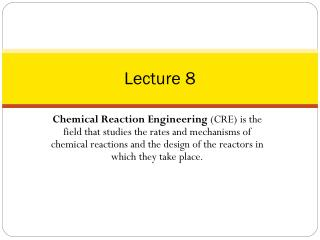Download PresentationLecture 8

Loading in 2 Seconds...

# Lecture 8 - PowerPoint PPT Presentation

Lecture 8. Chemical Reaction Engineering (CRE) is the field that studies the rates and mechanisms of chemical reactions and the design of the reactors in which they take place. Today’s lecture. Block 1: Mole Balances on PFRs and PBR Must Use the Differential Form Block 2: Rate LawsI am the owner, or an agent authorized to act on behalf of the owner, of the copyrighted work described.
Download Presentation## Lecture 8

An Image/Link below is provided (as is) to download presentation

Download Policy: Content on the Website is provided to you AS IS for your information and personal use and may not be sold / licensed / shared on other websites without getting consent from its author.While downloading, if for some reason you are not able to download a presentation, the publisher may have deleted the file from their server.

- - - - - - - - - - - - - - - - - - - - - - - - - - E N D - - - - - - - - - - - - - - - - - - - - - - - - - -
Presentation Transcript
1. Lecture 8 Chemical Reaction Engineering (CRE) is the field that studies the rates and mechanisms of chemical reactions and the design of the reactors in which they take place.

2. Today’slecture • Block 1: Mole Balances on PFRs and PBR • Must Use the Differential Form • Block 2: Rate Laws • Block 3: Stoichiometry • Pressure Drop: Liquid Phase Reactions: Pressure Drop does not affect the concentrations in liquid phase rx. Gas Phase Reactions: Epsilon not Equal to Zero d(P)/d(W)=.. Polymath will combine with d(X)/f(W)=..for you Epsilon = 0 and Isothermal P=f(W) Combine then Separate Variables (X,W) and Integrate

3. ReactorMoleBalances in terms of conversion X Batch t X CSTR W PFR PBR

4. Concentration Flow System: Gas Phase Flow System:

5. Pressure Drop in Packed Bed Reactors Note: Pressure dropdoes NOT affectliquidphasereactions MoleBalance: SampleQuestion: Analyze the following second order gas phasereaction that occursisothermally in a PBR: AB Must use the differential form of the molebalance to separate variables: Rate Law: Second order in A and irreversible:

6. Pressure Drop in Packed Bed Reactors Stoichiometry: Isothermal, T=T0 Combine: Need to find (P/P0) as a function of W (or V if you have a PFR)

7. Pressure Drop in Packed Bed Reactors ErgunEquation: Constant mass flow:

8. Pressure Drop in Packed Bed Reactors Variable Density Let

9. Pressure Drop in Packed Bed Reactors Where CatalystWeight Let

10. Pressure Drop in Packed Bed Reactors Wewilluse this form for singlereactions: Isothermal case

11. Pressure Drop in Packed Bed Reactors and or The two expressions are coupledordinary differential equations. Wecanonlysolvethemsimultaneouslyusing an ODE solversuch as Polymath. For the special case of isothermal operation and epsilon = 0, we can obtain an analytical solution. Polymathwillcombine the molebalance, rate law and stoichiometry.

12. PBR AB 1) MoleBalance: 2) Rate Law:

13. PBR

14. 1 P W

15. 2 CA W

16. 3 -rA W

17. 4 X W

18. 5 1.0 W

19. Example 1: Gas Phase Reaction in PBR for δ = 0 Gas Phase Reaction in PBR with δ = 0 (Polymath Solution) A + B  2C Repeat the previous one with equil molar feed of A and B and kA = 1.5dm9/mol2/kg/min α = 0.0099 kg-1 Find X at 100 kg

20. Example 1: Gas Phase Reaction in PBR for δ = 0 A + B  2C Case 1: Case 2:

21. Example 1: Gas Phase Reaction in PBR for δ = 0 1) MoleBalance: 2) Rate Law: 3) 4)

22. Example 1: Gas Phase Reaction in PBR for δ = 0

23. Example A + B → 2C

24. Example A + B → 2C

25. Example 2: Gas Phase Reaction in PBR for δ ≠ 0 Polymath Solution A + 2B  C is carried out in a packed bed reactor in which there is pressure drop.The fed is stoichiometric in A and B. Plot the conversion and pressure ratio y = P/P0 as a function of catalyst weight upto 100 kg. Additional Information kA = 6dm9/mol2/kg/min α = 0.02 kg-1

26. Example 2: Gas Phase Reaction in PBR for δ ≠ 0 A + 2B  C 1) MoleBalance: 2) Rate Law: 3) Stoichiometry:Gas, Isothermal

27. Example 2: Gas Phase Reaction in PBR for δ ≠ 0 4) 5) 6) 7) Initial values: W=0, X=0, y=1  W=100 Combine with Polymath. If δ≠0, polymath must be used to solve.

28. Example 2: Gas Phase Reaction in PBR forδ ≠ 0

29. Example 2: Gas Phase Reaction in PBR for δ ≠ 0

30. T = T0

31. Engineering Analysis

32. Engineering Analysis

33. Engineering Analysis

34. Pressure Change – Molar Flow Rate Isothermal: T = T0 Use for heat effects, multiple rxns

35. Heat Effects Isothermal Design Stoichiometry Rate Laws Mole Balance

36. End of Lecture 8